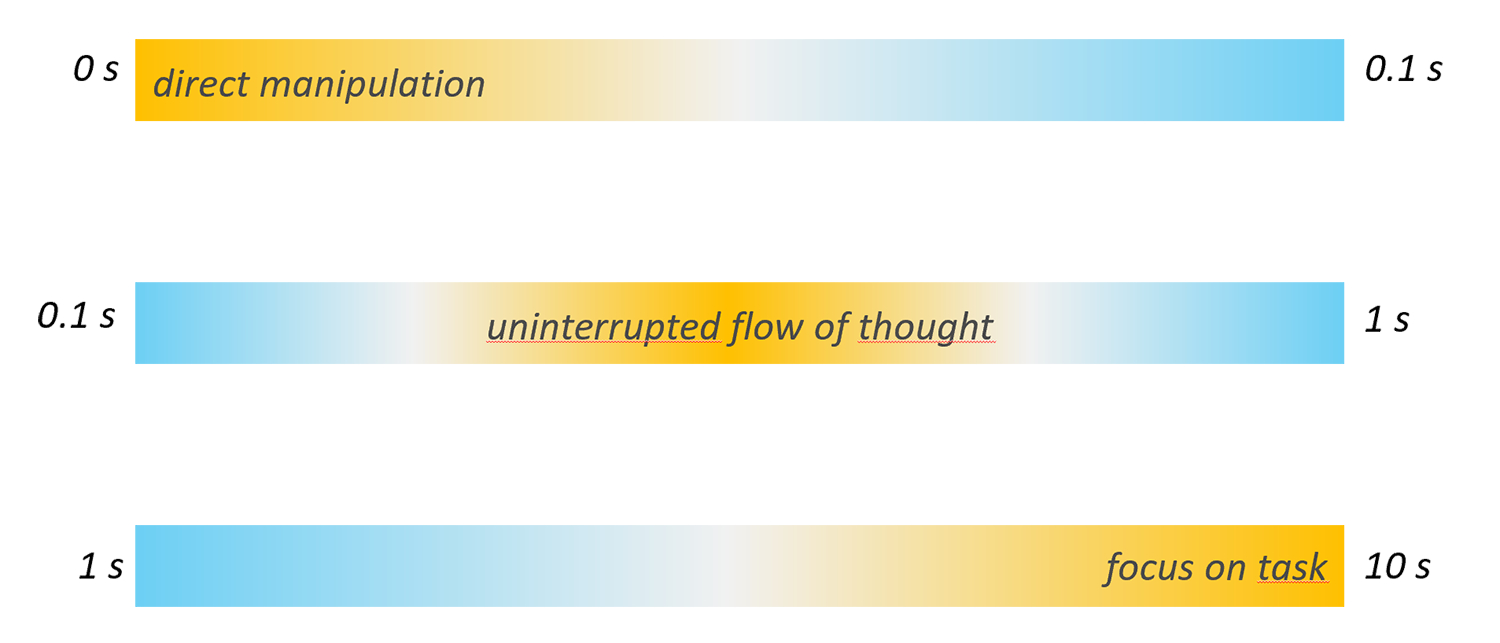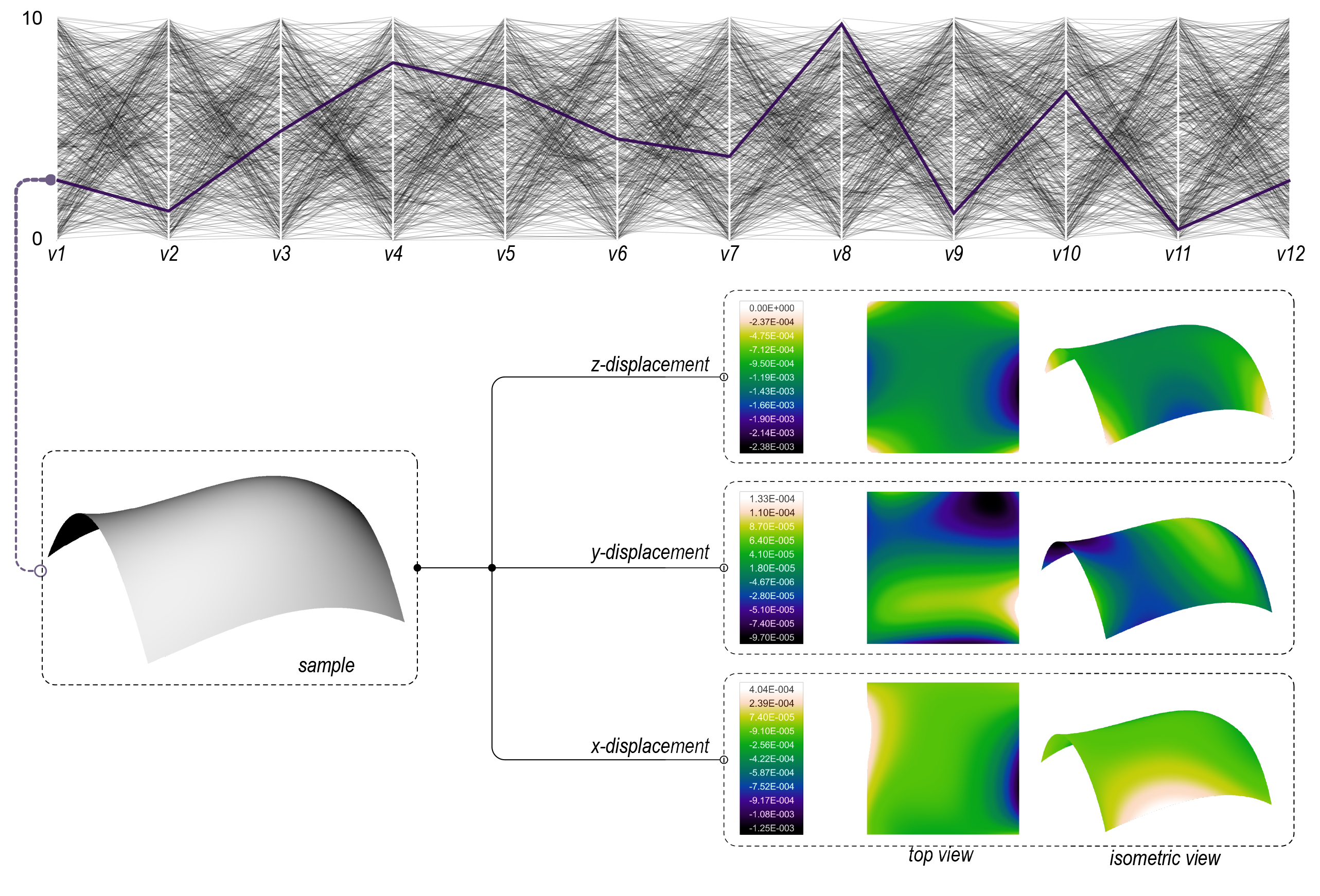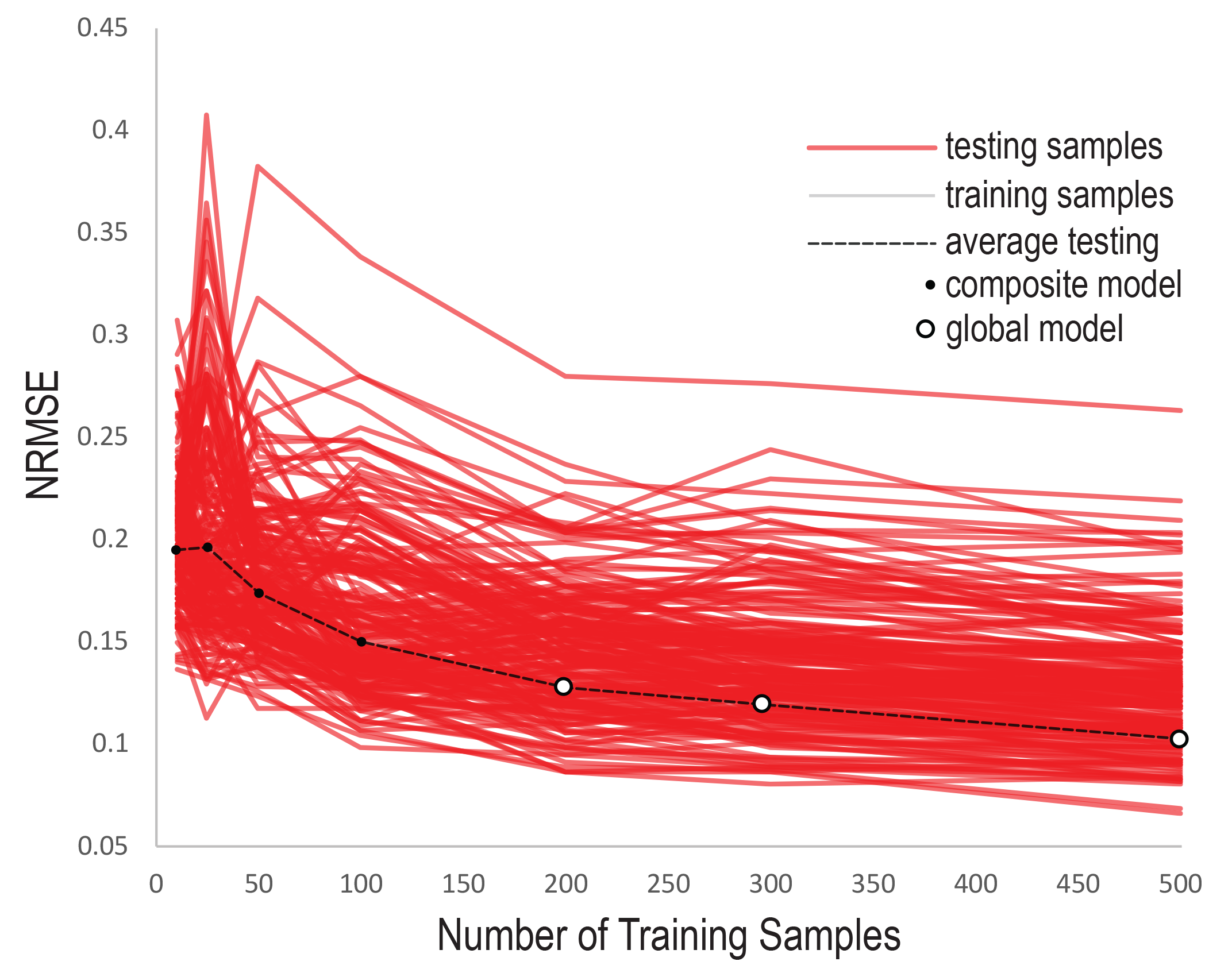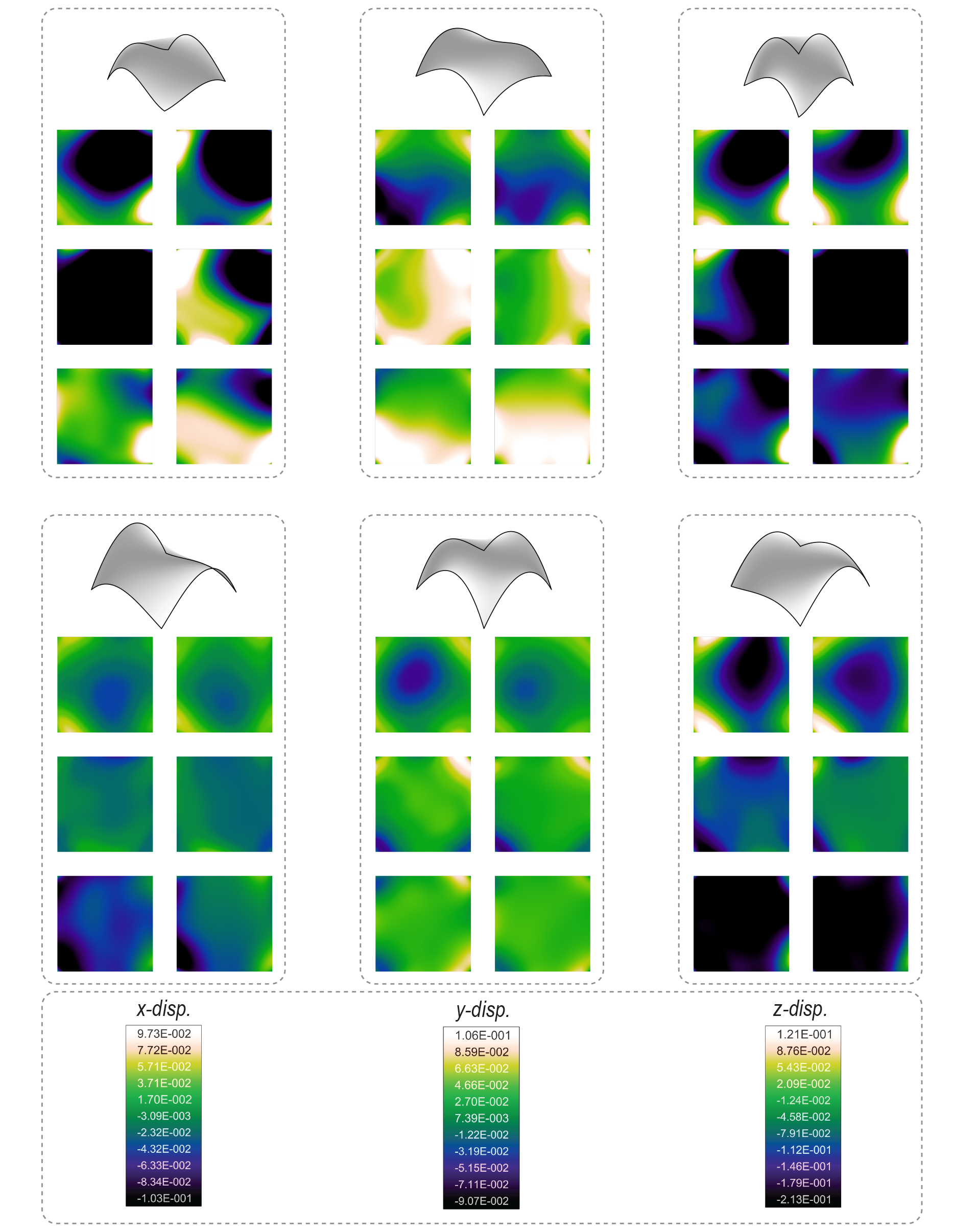## RD

04
learning surface fields
data | machine learning | surrogate modelling | 2018

Building simulations often return fields of information, e.g. solar radiation or stress fields, which serve as useful feedback for designers. However, those simulations may be time-consuming, limiting their effective use in iterative design processes. This research proposes to predict surface fields based in real-time using machine learning.
This page showcases our results on predicting displacement fields computed using isogeometric analysis on shells loaded with their own weight. I am currently extending the research to other types of simulation and different computational formats through the use of geometric deep learning.

With Caitlin Mueller

The machine learning models were built in C# using code available here.

This research proposes to add a layer of real-time structural feedback on a surface sculpted digitally, through the display of approximate deformation models in near-real-time, in order to foster feedback-based exploration of shells. While the models do not restrict the breadth of explorable shapes, visualizing how a structure deforms offers insight as to how one should tune its geometry. Analyzing a shell structure can be computationally intensive, in particular when advanced simulations, such as non-linear analyses, are to be considered. Slow analysis hinders the effective exploration of the design space, and it precludes designers from benefiting from real-time simulation feedback. This research uses machine learning algorithms to build fast, exploratory approximate models, or metamodels, which visualize a structure’s behavior as it is being shaped with near-instantaneous feedback and within a reasonable error margin.

During the conceptual design phase of a project, engineers investigate different possible geometries and iteratively converge to a solution based on analysis feedback and other, less quantifiable objectives. Unfortunately, analyzing a structure may be a computationally intensive process, in particular when complex phenomena, such as buckling, ought to be considered. It may also be non-trivial or slower than required because of interoperability issues. This prevents designers from exploring the space of possible geometries with real-time performance feedback. To address this, previous work has focused on building surrogate models for the prediction of single scalar outputs (Tseranidis et al., 2016; Forrester et al. 2008). Often, however, visualizing how a structure deforms or displaces offers more insight than single performance metrics as to how and where one should tune the geometry. This research uses portable regression models, or metamodels, in order to predict the displacement shape of a structure in nearly-real-time. With the built metamodel, the displaced configuration of a structure can be displayed nearly instanteneously as the designer sculpts it in the CAD environment to tune its behavior.

The video belows shows a trained model used in Rhino 3D to predict the x-,y-, and z-displacement fields of a shell as it is being modelled. For comparison, the exact analysis would take on average 4 seconds to compute.

## Motivation: need for speed

With the increasing integration of simulation tools within CAD environments, we have the opportunity to use simulations as performance feedback to guide our design choices. Unfortunately, these simulations are often time-consuming, which hinders their use for design space exploration. Human-computer research interaction research has shown that sluggish response times lead to a substantial loss of productivity (Miller, 1968), and that software systems should operate fast enough such that the user can maintain an uninterrupted flow of thought or, better yet, feel like they are directly manipulating the interface.For optimal exploration capabilities, we should be aiming for models that provide feedback in less 0.1 second. More often than not, simulations will take longer to run than this limit, which whill hinder effective design space exploration One way to get real-time feedback is thus to use surrogate models based on a limited set of simulation data points or samples. (Nielsen, 1993)

This research seeks to replace slow exact analysis with fast, approximate models that provide results which can be effectively used by designers.

## Sampling

Surrogate modelling differs from traditional data-based regression methods in that the data is not collected from real-world processes. Instead, it is produced by numerical simulations, and the amount of data generated is only limited by the time necessary to run the simulations. Theoretically, it is possible to obtain as much data as one would need. However, in practice, time constraints play an important role, and it is thus paramount to sample to space effectively with as few points as possible. In this work, the data points are generated using a latin hypercube sampling.

First, the input variables are sampled in order to generate a collection of vectors of input variables, which define the sampled surface structures. These structures are then analyzed using isogeometric analysis to simulate the displacement fields of the control points in the x, y, and z directions.Sampling methodology: the feature space is sampled using random uniformly distributed data points. The parallel coordinate plot represent a dataset of 500 samples (each line corresponds to an individual sample, and each vertical axis to a design variable). A specific example is highlighted in purple: its corresponding geometry is used as analysis input for isogeometric analysis, which determines the deformed state of the surface and whose results can be used for visualization in a design environment.

These displacements can be visualized using color maps, which indicate how and where the displacements occur. Designers use these color maps as an indication of the surface behavior, and an effective approximation system will produce color maps that ‘look’ similar to the exact ones.

## Training

Once the data is generated, it used to train multiple types of regression models (neural network, random forest, ridge regression) with different hyperparamter values. The best one is selected using cross-validation (based on MSE). Unsurprisingly, the quality of the model, as measured by the normalized RMSE for example, is highly dependent on the number of samples, as shown by the image below.Evolution of NRMSE on testing model with 50 random samples as a function of number of samples (10, 25, 50, 100, 200, 300, and 500) used to build the regression model. Each red line corresponds to the error of a unique output variable (192); the dashed black line is the NRMSE averaged over all of the output variables. A black dot corresponds to a composite overall best model (model without parameter sharing across output targets), whereas a white corresponds to a global overall best model.

## Results

The image below demonstrates the visual predictions given by the model obtained with 200 training samples on 6 random test samples. The relatively low NRMSE is translated into color maps that ‘look’ similar. Of course, the net result of the trained metamodel is a live visualization of the displacement fields occurring in the structure under the given loads as the geometry is sculpted digitally.Results obtained on 6 random testing samples using the model built using 200 training samples. The 6 rectangles at the top each represent one testing sample, with a perspective view of its corresponding geometry, followed by two vertical trios of color maps (left: exact displacement map calculated using IGA; right: predicted displacement map; top to bottom: z-, y-, and x-displacement). If the model performed perfectly, the two columns of color maps for each design would look identical. The magnitudes of the x-, y-, and z-displacements are indicated in the bottom rectangle.

The work demonstrates the potential of ML regression techniques for predicting fields resulting from simulations. When integrated in a CAD environment, these models allow for near-real-time feedback on the impact of geometric changes on the behavior of structure. SO far, this research serves as a proof of concept, and there are many opportunities for future research.

Preliminary results from this research were published at the IASS 2018 symposium in Boston. The project is ongoing.